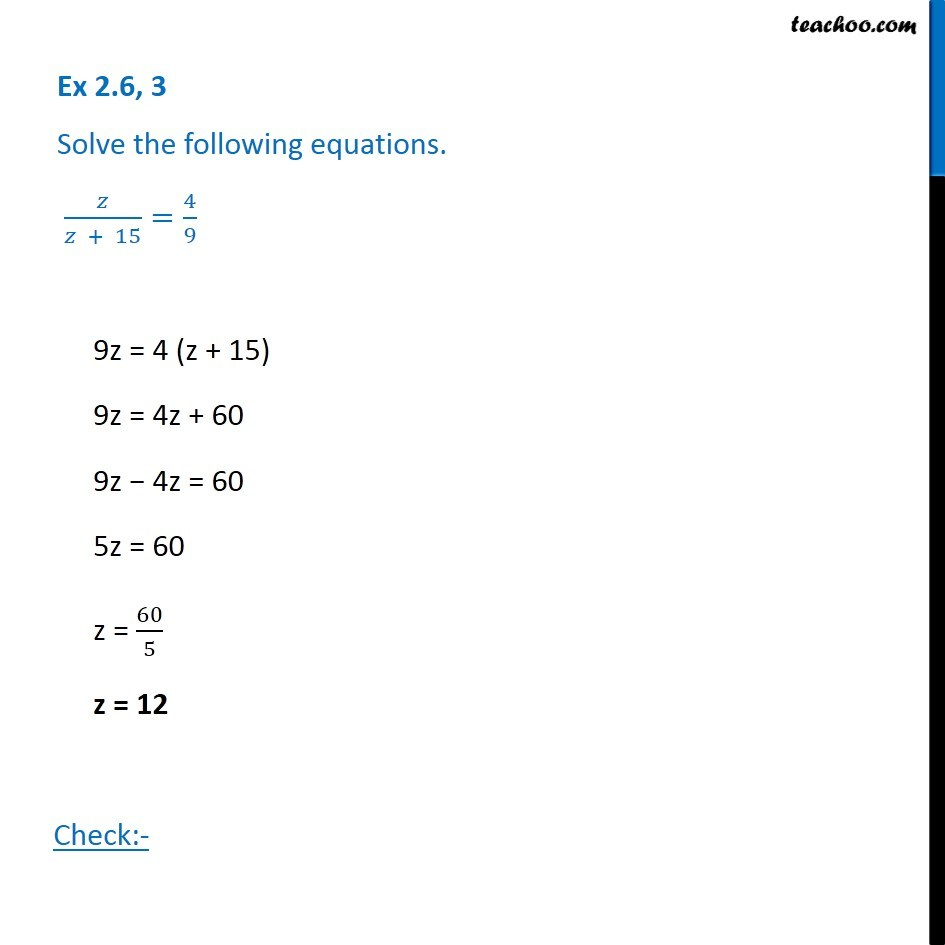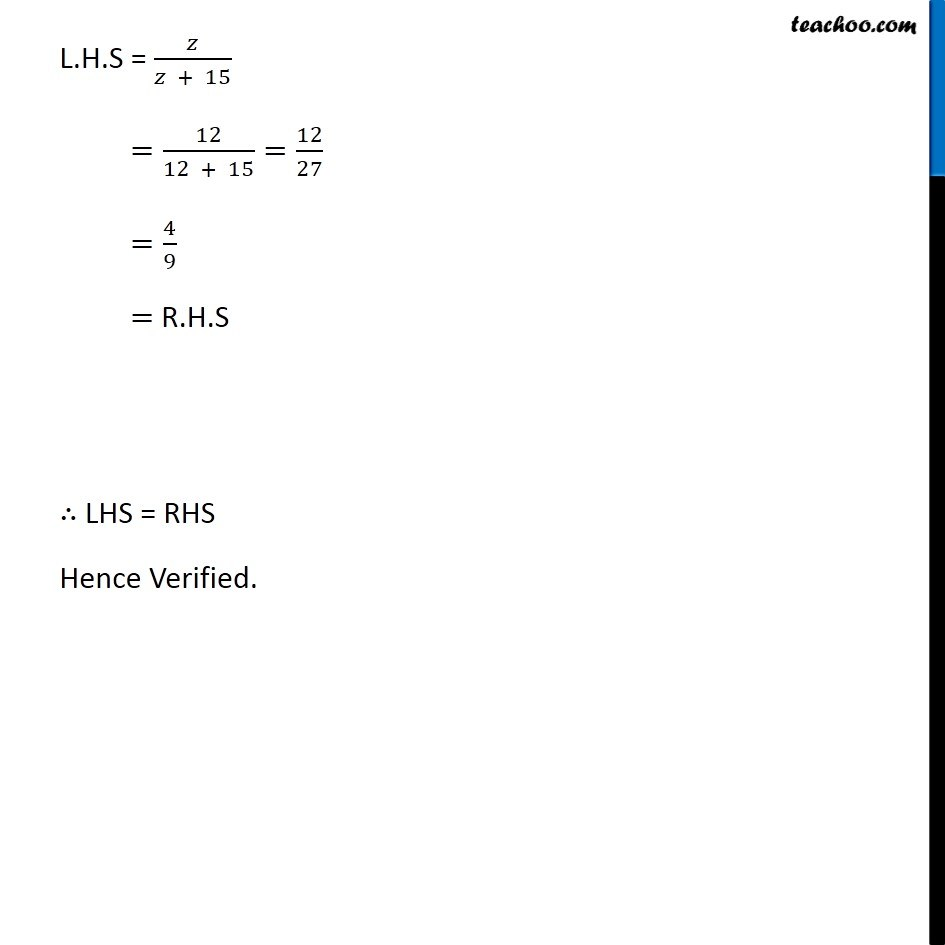Solving difficult equations - Word Problems

Chapter 2 Class 8 Linear Equations in One Variable
Serial order wiseLearn in your speed, with individual attention - Teachoo Maths 1-on-1 Class

### Transcript

Question 3 Solve the following equations. 𝑧/(𝑧 + 15)=4/99z = 4 (z + 15) 9z = 4z + 60 9z − 4z = 60 5z = 60 z = 60/5 z = 12 Check:- L.H.S = 𝑧/(𝑧 + 15) =12/(12 + 15)=12/27 =4/9 = R.H.S ∴ LHS = RHS Hence Verified.